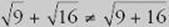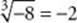﻿ ﻿SQUARES AND SQUARE ROOTS - Basic Arithmetic - TOPICS IN ARITHMETIC - SAT SUBJECT TEST MATH LEVEL 1

## TOPICS IN ARITHMETIC## CHAPTER 2Basic Arithmetic### SQUARES AND SQUARE ROOTS

The exponent that appears most often on the Math 1 test is 2. Although a2 can be read “a to the second,” it is usually read “a squared.” You will see 2 as an exponent in many formulas. For example:

• A = s 2 (the area of a square)

• A = πr 2 (the area of a circle)

• a2 + b2 = c 2 (the Pythagorean theorem)

• x2 - y 2 = (x - y)(x + y) (factoring the difference of two squares)

Numbers that are the squares of integers are called perfect squares. You should recognize at least the squares of the integers from 0 through 15.Of course if you need to evaluate 132, you can use your calculator. However, it is often helpful to recognize these perfect squares. That way, if you see 169, you will immediately think “that is 132.”

Two numbers, 5 and –5, satisfy the equation x2 = 25. The positive one, 5, is called the square root of 25 and is denoted by the symbol. Clearly, each perfect square has a square root:,,, and. However, it is an important fact that every positive numberhas a square root.

Key Fact A12

For any positive number a, there is a positive number b that satisfies the equation b2= a. That number, b, is called the square root of a and is written. So, for any positive number a,.

Key Fact A13

For any positive numbers a and b:For example,and.

Don”t Get ConfusedFor example:The expression a3 is often read “a cubed.” Numbers that are the cubes of integers are called perfect cubes. You should memorize the perfect cubes in the following table.The only other powers you should recognize immediately are the powers of 2 up to 210.In the same way that we writeto indicate that b2 = a:

• We writeto indicate that b3 = a and call b the cube root of a.

• We writeto indicate that b4 = a and call b the fourth root of a.

• For any integer n2, we writeto indicate that bn = a and call b the nth root of a.

For example:because 53 = 125.because (–2)3 = –8.because 210 = 1024.

Note thatis undefined because there is no real number x such that x 2 = –64. If you enteron your calculator, you will get an error message. (In Chapter 17, you will read about the imaginary unit i and will review the fact thatis 8i.)

Key Fact A14

For any real number a and integer n2:

If n is odd, thenis the unique real number x that satisfies the equation xn= a.

If n is even and a is positive, thenis the unique positive number x that satisfies the equation xn= a.

We can now expand our definition of exponents to include fractions.

Key Fact A15

For any positive number b and positive integers n and m with n2:For example:The laws of exponents, listed in KEY FACT A11, are equally valid if any of the exponents are fractions. For example:﻿